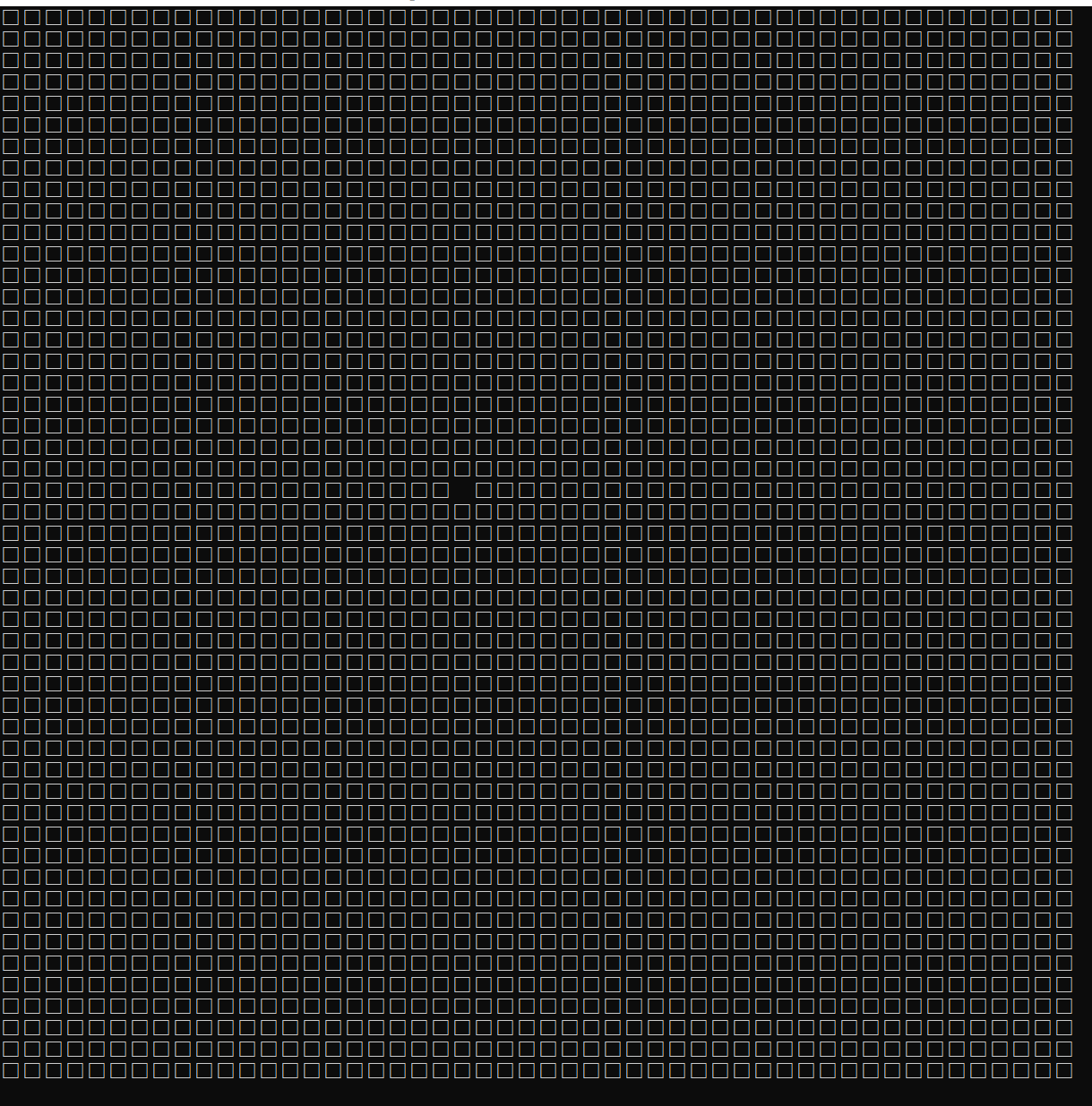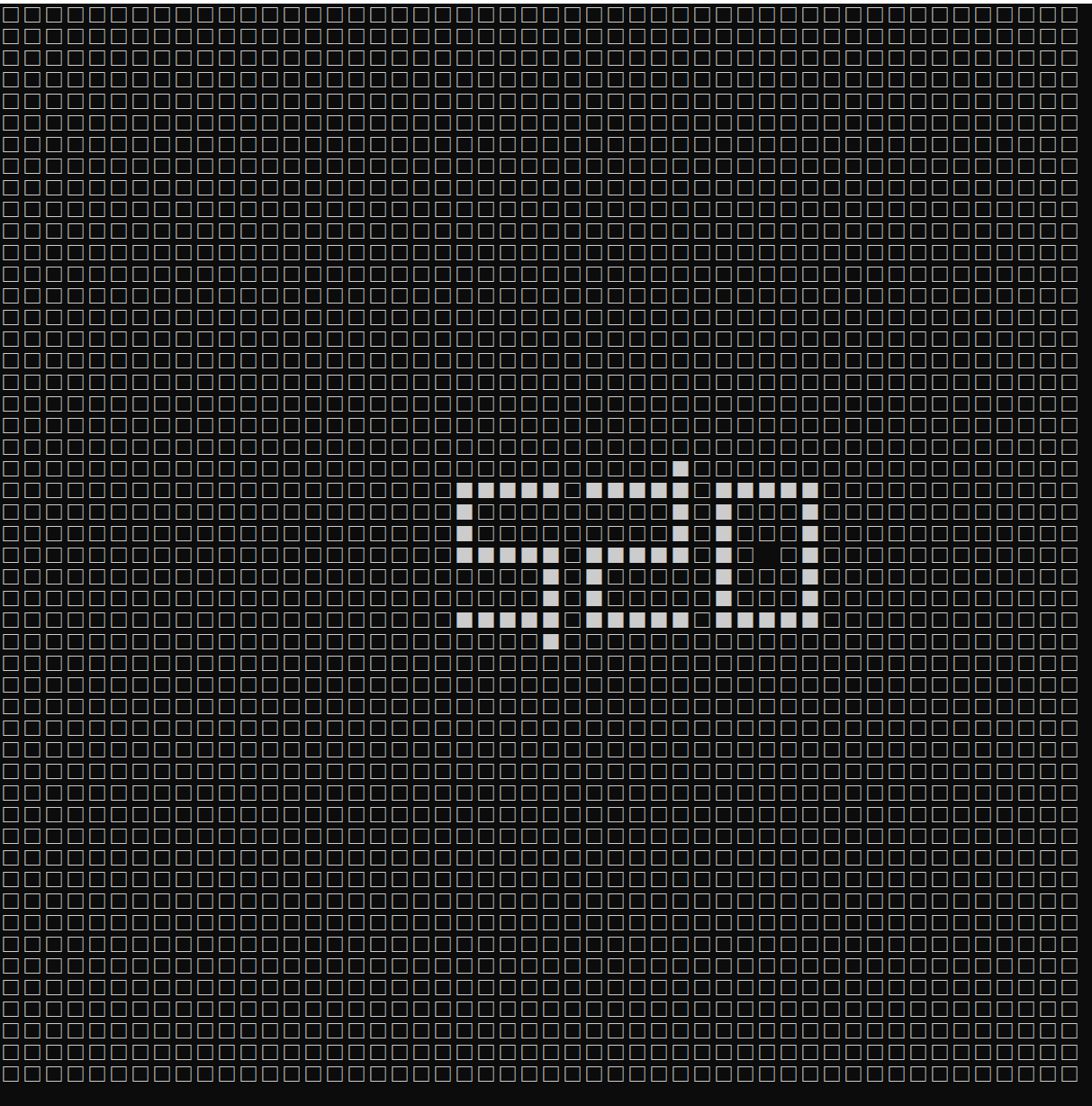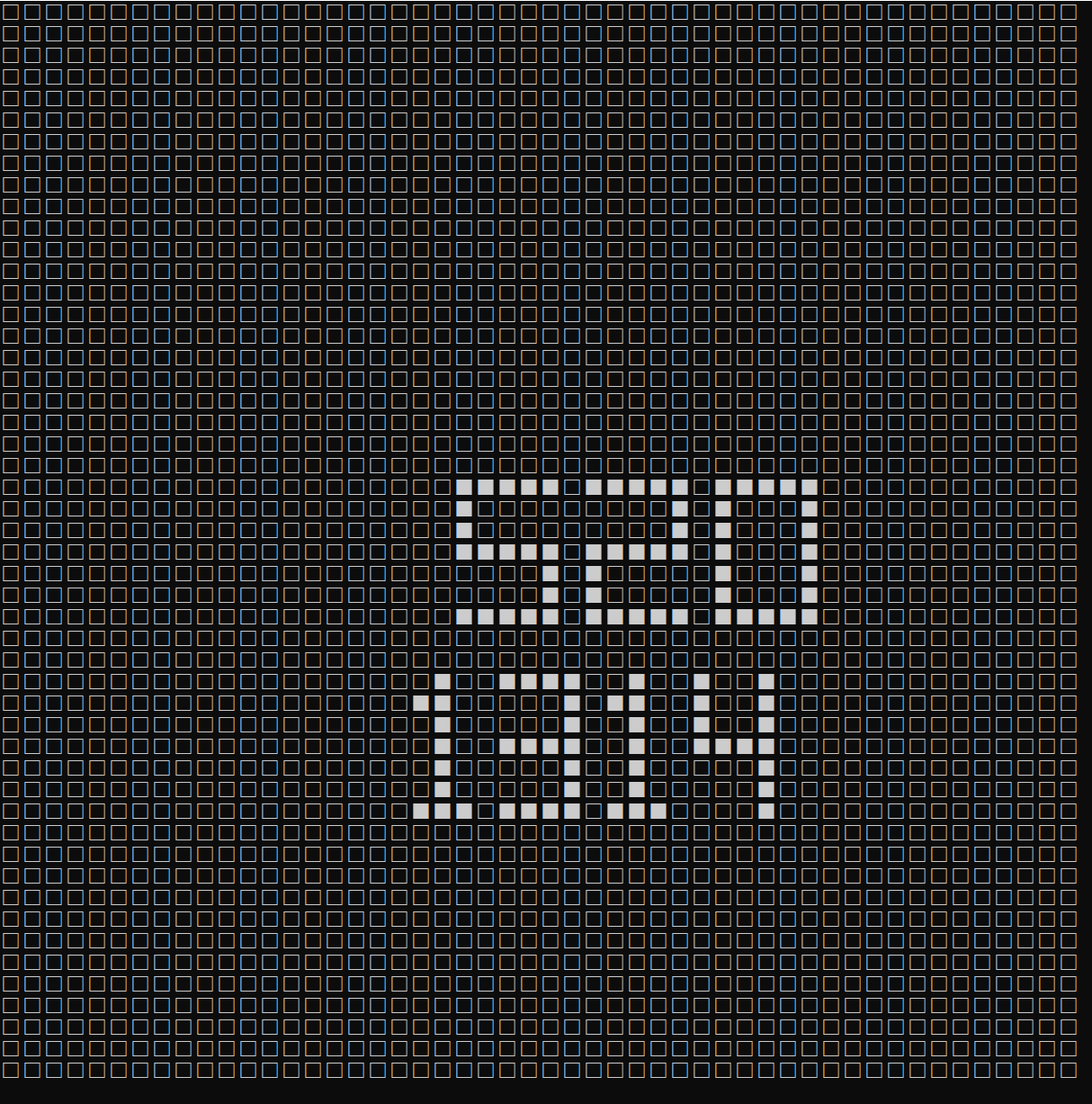• 虽然已经学习了一个学期的c语言，但这也是我第一次写博客，之前一直没时间或者是觉得自己那个实在没什么好写的，最近我突然性情大发，生了想要好好写一篇博客的心思，也算是记录一下自己的进步。 今天要讲的是我最近...
文章目录前言一、程序介绍二、使用步骤1.引入库2.定义静态变量，地图大小3.画出图像（地图）4.控制移动的子函数5.以及最后的主函数总结

前言
虽然已经学习了一个学期的c语言，但这也是我第一次写博客，之前一直没时间或者是觉得自己那个实在没什么好写的，最近我突然性情大发，生了想要好好写一篇博客的心思，也算是记录一下自己的进步。
今天要讲的是我最近写的一个我还算满意的小程序，因为放了寒假，闲在家里，就生了写小程序的心思，正好我女朋友还没放假，趁现在我写了个代码给我女朋友。就是这个c语言写的方格绘图（随便取的名字）小程序

一、程序介绍
方格绘图小程序是只有黑白方框，像一个个小像素点一般的，远远看上去像一副图片，通过wasd来操控上下左右，c键来画，x键来清除错误的白格子。
成品图
一开始然后开始绘画，如果不小心画错了也无妨最后成果，女朋友很满意二、使用步骤
1.引入库
代码如下：
#include<stdio.h>
#include<stdlib.h>
#include<conio.h>

2.定义静态变量，地图大小
代码如下：
int static o = 0, p = 0,med=0,fad=0;
int map = {
{3},
};//地图大小的划定

3.画出图像（地图）
代码如下：
void drawmap()//绘图
{
int i, j;
for (i = 0; i < 50; i++)
{
for (j = 0; j < 50; j++)
{
switch (map[i][j])
{
case 0:printf("□"); break;
case 1:printf("■"); break;
case 3:printf("  "); break;
default:break;
}
}printf("\n");
}
}

4.控制移动的子函数
代码如下：
void  move(int n,int m)//移动方格的函数
{
if (o < 50 && p < 50)
{
map[o][p] = med;
fad = map[o + n][p + m];
map[o + n][p + m] = 3;
o = o + n;
p = p + m;
}
else
{
printf("超出边界，重新选择移动路径：\n");//修复可能存在的bug
}
}

5.以及最后的主函数
代码如下：
int main()
{

char c;

for (;;)
{
system("cls");
drawmap();
c = _getch();
switch (c)//改进了其操作方式使其操作更简单一点
{
case 'a':move(0, -1); break;
case 'w':move(-1, 0); break;
case 's':move(1, 0); break;
case 'd':move(0, 1); break;
case 'c':map[o][p] = 1;med= map[o][p]; break;//变为白方格
case 'x':map[o][p] = 0;med = map[o][p]; break;//变为黑方框
default:break;
}
}
}


总结
最后的全部代码，放在这里，想要的自取。博主用的是visual studio 2019
//小王同学花了一下午写的画图小程序
#include<stdio.h>
#include<stdlib.h>
#include<conio.h>
int static o = 0, p = 0,med=0,fad=0;
int map = {
{3},
};//地图大小的划定
void drawmap()//绘图
{
int i, j;
for (i = 0; i < 50; i++)
{
for (j = 0; j < 50; j++)
{
switch (map[i][j])
{
case 0:printf("□"); break;
case 1:printf("■"); break;
case 3:printf("  "); break;
default:break;
}
}printf("\n");
}
}
void  move(int n,int m)//移动方格的函数
{
if (o < 50 && p < 50)
{
map[o][p] = med;
fad = map[o + n][p + m];
map[o + n][p + m] = 3;
o = o + n;
p = p + m;
}
else
{
printf("超出边界，重新选择移动路径：\n");//修复可能存在的bug
}
}
int main()
{

char c;

for (;;)
{
system("cls");
drawmap();
c = _getch();
switch (c)//改进了其操作方式使其操作更简单一点
{
case 'a':move(0, -1); break;
case 'w':move(-1, 0); break;
case 's':move(1, 0); break;
case 'd':move(0, 1); break;
case 'c':map[o][p] = 1;med= map[o][p]; break;//变为白方格
case 'x':map[o][p] = 0;med = map[o][p]; break;//变为黑方框
default:break;
}
}
}

差不多，我的第一个博客就写到这了，以后有情况多多补充！冲冲冲！加油！


展开全文• C语言画图 计算机 .编程 C语言画图 计算机 .编程
• C语言 画图颜色管理实例C语言 画图颜色管理实例C语言 画图颜色管理实例C语言 画图颜色管理实例C语言 画图颜色管理实例
• 用graphics函数坦克图画：#include"graphics.h"#include"conio.h"void Tanke(int x,int y,int R,int TColor,int FColor){int i,j,d,t;d=R/4;t=d*0.6;setcolor(TColor);/*------------------------------------------...
用graphics函数坦克图画：#include"graphics.h"#include"conio.h"void Tanke(int x,int y,int R,int TColor,int FColor){int i,j,d,t;d=R/4;t=d*0.6;setcolor(TColor);/*--------------------------------------------*/line(x-R,y,x+R-t,y);line(x-R,y,x-R+d,y+2*d);line(x-R+d,y+2*d,x+3*d,y+2*d);line(x+3*d,y+2*d,x+R-t,y);/*--------------------------------------------*/line(x-R,y,x-R+t,y-t);line(x-R+t,y-t,x-2*d,y-t);line(x-2*d,y-t,x-2*d+t,y-d-t);line(x-2*d+t,y-d-t,x-3*d,y-d-t);line(x-3*d,y-d-t,x-3*d+t,y-2*d);line(x-3*d+t,y-2*d,x+R-t,y-2*d);line(x+R-t,y-2*d,x+R-t,y);/*-------------------------------------------*/line(x-3*d,y-d-t,x-2*d,y-t);/*-------------------------------------------*/line(x-R+d-t,y+2*(d-t),x+3*d+R*0.04,y+2*(d-t));for(i=0;i<2*(R/d-1);i++)line(x-3*d+i*d,y+2*(d-t),x-3*d+i*d,y+2*d);/*-------------------------------------------*/setfillstyle(1,TColor);bar(x-d*1.2,y-d*0.6,x+d*2,y-d*0.2);line(x-d*1.2,y-d*0.6,x-d*0.6,y-d*1.8);line(x-d*0.6,y-d*1.8,x+d*2.4,y-d*1.8);line(x+d*2.4,y-d*1.8,x+d*2,y-d*0.6);line(x+d*2.4,y-d*1.8,x+d*2.4,y-d*1.4);line(x+d*2,y-d*0.2,x+d*2.4,y-d*1.4);/*-------------------------------------------*/sector(x+d*0.6,y-d,0,180,d*1.2,d*1.4);/*-------------------------------------------*/for(i=0;iline(x+d*0.2,y-d*1.8+i,x-d*2.5,y-d*1.8+i);setfillstyle(1,FColor);bar(x-d*3,y-d*1.9,x-d*2.3,y-d*1.3); /*炮头(红色)*/}main(){int gd=DETECT,gm;initgraph(&gd,&gm,"");setcolor(0);Tanke(300,200,50,WHITE,RED);getch();closegraph();}
展开全文• c语言画图的代码，代码类，简单的画图。希望对大家有借鉴学习之用。。
• c语言画图及小动画制作（graphics.h函数入门）
• c语言画图源代码包含了用C语言实现的多类图像的绘画以及其源代码。
• 这是c语言画图函数库-EasyX库.rar，希望对大家有帮助
• 计算机图形学 C语言 画图程序 支持鼠标 源程序 在学校写的课程设计。
• C语言画图及小动画C语言画图及小动画制作(graphisc.h函数入门)制作(grC语言画图及小动画制作(graphisc.h函数入门)aphisc.h函数入门)
• ## 用C语言画图

万次阅读 多人点赞 2017-04-07 20:55:06
在没有桌面和图形文件的系统界面，可以通过C语言的编程来实现在黑色背景上画图！ 用下面的代码，在需要的地方（有注释）适当修改，就能画出自己喜欢的图形！ PS：同样要编译运行后才能出效果。 #include #include ...

framebuffer(帧缓冲)。帧的最低数量为24（人肉眼可见）（低于24则感觉到画面不流畅）。显卡与帧的关系：由cpu调节其数据传输速率来输出其三基色的配比。三基色：RGB（红绿蓝）。
在没有桌面和图形文件的系统界面，可以通过C语言的编程来实现在黑色背景上画图！
用下面的代码，在需要的地方（有注释）适当修改，就能画出自己喜欢的图形！
PS：同样要编译运行后才能出效果。

#include <stdio.h>
#include <sys/mman.h>
#include <fcntl.h>
#include <linux/fb.h>
#include <stdlib.h>

#define	 RGB888(r,g,b)	 ((r & 0xff) <<16 | (g & 0xff) << 8 | (b & 0xff))
#define	 RGB565(r,g,b)	 ((r & 0x1f) <<11 | (g & 0x3f) << 5 | (b & 0x1f))

int main()
{
int fd = open("/dev/fb0", O_RDWR);
if(fd < 0){
perror("open err. \n");
exit(EXIT_FAILURE);
}
struct fb_var_screeninfo info;

if ( (ioctl(fd, FBIOGET_VSCREENINFO, &info)) < 0){
perror("ioctl err. \n");
exit(EXIT_FAILURE);
}

printf("xres: %d\n", info.xres);
printf("yres: %d\n", info.yres);
printf("bits_per_pixel: %d\n", info.bits_per_pixel);

size_t len = info.xres*info.yres*info.bits_per_pixel >> 3;

perror("mmap err. \n");
exit(EXIT_FAILURE);
}

int i,j;
for(i=0; i<768; i++){						//不同的电脑和系统像素不同，可以根据情况改变1366*768两个数的大小。
for(j=0; j<1366; j++)
//*(addr+i*1366+j) = RGB888(255, 0, 0);		//此处为画图位置，根据不同的数学公式，就可以给1366*768个像素上色。形成不一样的颜色！
*(addr+i*1366+j) = RGB565(255, 0, 0);		//此为两种规格的像素。3个数分别带面红绿蓝的色彩深度。
}
printf("hello main \n");

if(ret < 0){
perror("munmap err. \n");
exit(EXIT_FAILURE);
}

return 0;
}


展开全文• 图形和图像函数包含在graphics.h里面 (一) 像素函数 56. putpiel() 画像素点函数 57. getpixel()返回像素色函数 (二) 直线和线型函数 58. line() 画线函数 59. lineto() 画线函数 60. linerel() 相对画线...
• 呵呵昨天花了一个圆，想画个太极图，我知道没啥技术含量，但是挺有意思的，希望各位看官不要鄙视我不务正业，画完此图，不再做这些事情。 　先展示下画出来的图像的情况，因为不支持pgm格式的图像，所以我用的 QQ......

# c语言画图c语言 订阅